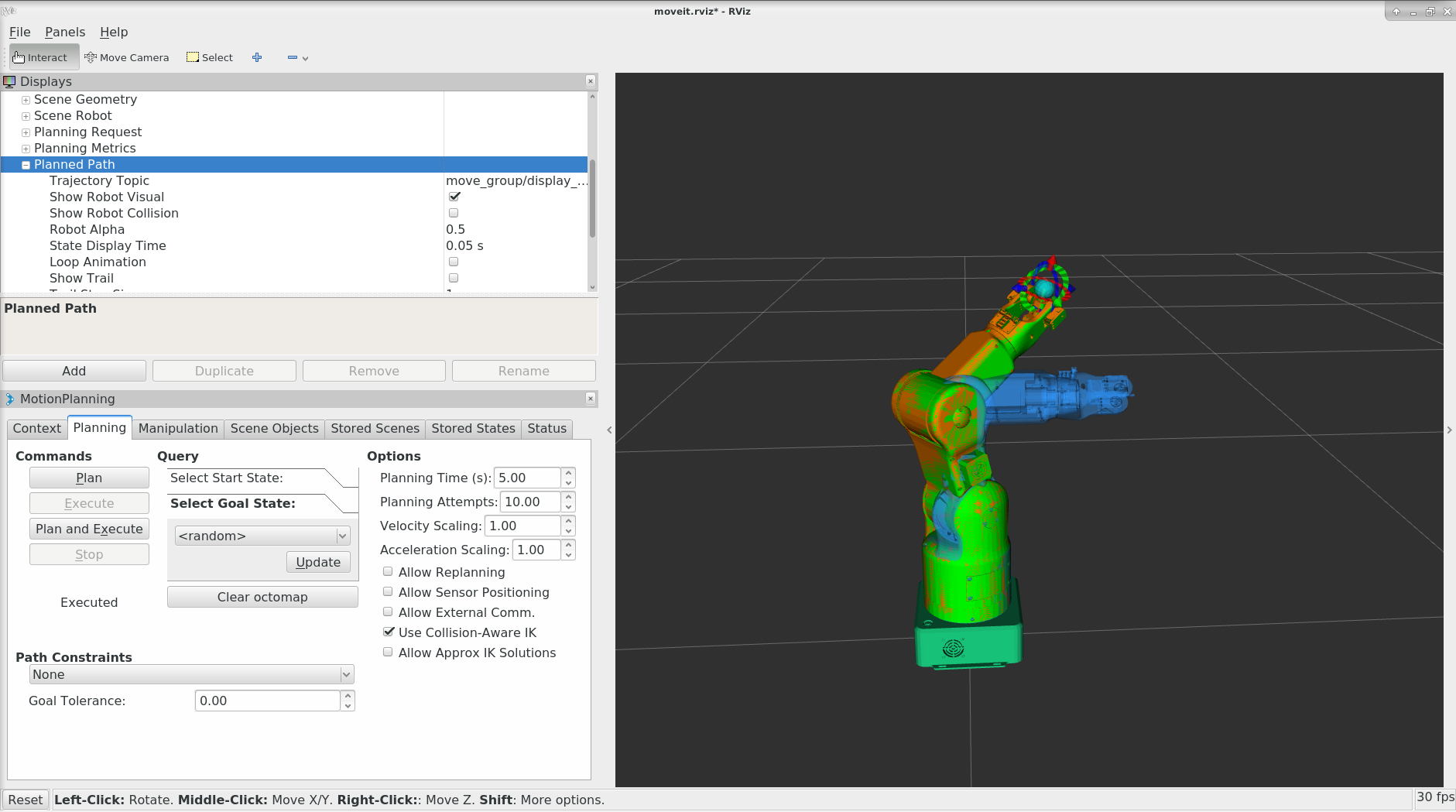# 3.6 笛卡尔空间规划¶#include <moveit/move_group_interface/move_group_interface.h>

int main(int argc, char** argv)
{
ros::init(argc, argv, "move_group_interface_tutorial");
ros::NodeHandle node_handle;
ros::AsyncSpinner spinner(1);
spinner.start();

// BEGIN_TUTORIAL
//
// Setup
// ^^^^^
//
// MoveIt! operates on sets of joints called "planning groups" and stores them in an object called
// the JointModelGroup. Throughout MoveIt! the terms "planning group" and "joint model group"
// are used interchangably.
static const std::string PLANNING_GROUP = "gauss_arm";

// The :move_group_interface:MoveGroup class can be easily
// setup using just the name of the planning group you would like to control and plan for.
moveit::planning_interface::MoveGroupInterface move_group(PLANNING_GROUP);

// Getting Basic Information
// ^^^^^^^^^^^^^^^^^^^^^^^^^
//
// We can print the name of the reference frame for this robot.
ROS_INFO_NAMED("tutorial", "Reference frame: %s", move_group.getPlanningFrame().c_str());

// We can also print the name of the end-effector link for this group.

// Start the demo

// Planning to a Pose goal
// ^^^^^^^^^^^^^^^^^^^^^^^
// We can plan a motion for this group to a desired pose for the
// end-effector.
geometry_msgs::Pose target_pose1;
// target_pose1.orientation.w = 1.0;
// target_pose1.orientation.x = 0;
// target_pose1.orientation.y = 0;
// target_pose1.orientation.z = 0;
target_pose1.orientation = tf::createQuaternionMsgFromRollPitchYaw(0,0,0);

target_pose1.position.x = (double)276/1000;
target_pose1.position.y = 0;
target_pose1.position.z = (double)435/1000;
move_group.setPoseTarget(target_pose1);

// Now, we call the planner to compute the plan and visualize it.
// Note that we are just planning, not asking move_group
// to actually move the robot.
moveit::planning_interface::MoveGroupInterface::Plan my_plan;

bool success = (move_group.plan(my_plan) == moveit::planning_interface::MoveItErrorCode::SUCCESS);

ROS_INFO_NAMED("tutorial", "plan 1 (pose goal) %s", success ? "" : "FAILED");

// Moving to a pose goal
// ^^^^^^^^^^^^^^^^^^^^^
//
// Moving to a pose goal is similar to the step above
// except we now use the move() function. Note that
// the pose goal we had set earlier is still active
// and so the robot will try to move to that goal. We will
// not use that function in this tutorial since it is
// a blocking function and requires a controller to be active
// and report success on execution of a trajectory.

/* Uncomment below line when working with a real robot */
move_group.move();

ros::shutdown();
return 0;
}